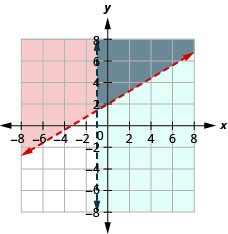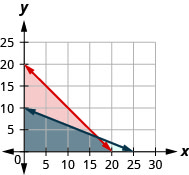# 4.8E：练习

•• OpenStax
• OpenStax
$$\newcommand{\vecs}{\overset { \rightharpoonup} {\mathbf{#1}} }$$ $$\newcommand{\vecd}{\overset{-\!-\!\rightharpoonup}{\vphantom{a}\smash {#1}}}$$$$\newcommand{\id}{\mathrm{id}}$$ $$\newcommand{\Span}{\mathrm{span}}$$ $$\newcommand{\kernel}{\mathrm{null}\,}$$ $$\newcommand{\range}{\mathrm{range}\,}$$ $$\newcommand{\RealPart}{\mathrm{Re}}$$ $$\newcommand{\ImaginaryPart}{\mathrm{Im}}$$ $$\newcommand{\Argument}{\mathrm{Arg}}$$ $$\newcommand{\norm}{\| #1 \|}$$ $$\newcommand{\inner}{\langle #1, #2 \rangle}$$ $$\newcommand{\Span}{\mathrm{span}}$$ $$\newcommand{\id}{\mathrm{id}}$$ $$\newcommand{\Span}{\mathrm{span}}$$ $$\newcommand{\kernel}{\mathrm{null}\,}$$ $$\newcommand{\range}{\mathrm{range}\,}$$ $$\newcommand{\RealPart}{\mathrm{Re}}$$ $$\newcommand{\ImaginaryPart}{\mathrm{Im}}$$ $$\newcommand{\Argument}{\mathrm{Arg}}$$ $$\newcommand{\norm}{\| #1 \|}$$ $$\newcommand{\inner}{\langle #1, #2 \rangle}$$ $$\newcommand{\Span}{\mathrm{span}}$$$$\newcommand{\AA}{\unicode[.8,0]{x212B}}$$

## 练习成就完美

1。 $$\left\{\begin{array} {l} 3x+y>5\\2x−y\leq 10\end{array}\right.$$

$$(3,−3)$$
$$(7,1)$$

2。 $$\left\{\begin{array} {l} 4x−y<10\\−2x+2y>−8\end{array}\right.$$

$$(5,−2)$$
$$(−1,3)$$

ⓐ false ⓑ 真的

3。 $$\left\{\begin{array} {l} y>\frac{2}{3}x−5\\x+\frac{1}{2}y\leq 4\end{array}\right.$$

$$(6, −4)$$
$$(3, 0)$$

4。 $$\left\{\begin{array} {l} y<\frac{3}{2}x+3\\ \frac{3}{4}x−2y<5\end{array}\right.$$

$$(−4,−1)$$
$$(8, 3)$$

ⓐ false ⓑ 真的

5。 $$\left\{\begin{array} {l} 7x+2y>14\\5x−y\leq 8\end{array}\right.$$

$$(2, 3)$$
$$(7, −1)$$

6。 $$\left\{\begin{array} {l} 6x−5y<20\\−2x+7y>−8 \end{array}\right.$$

$$(1, −3)$$
$$(−4, 4)$$

ⓐ false ⓑ 真的

7。 $$\left\{\begin{array} {l} y\leq 3x+2\\y>x−1\end{array}\right.$$

8。 $$\left\{\begin{array} {l} y<−2x+2\\y\geq −x−1\end{array}\right.$$9。 $$\left\{\begin{array} {l} y<2x−1\\y\leq −\frac{1}{2}x+4\end{array}\right.$$

10。 $$\left\{\begin{array} {l} y\geq −\frac{2}{3}x+2\\y>2x−3\end{array}\right.$$11。 $$\left\{\begin{array} {l} x−y>1\\y<−\frac{1}{4}x+3\end{array}\right.$$

12。 $$\left\{\begin{array} {l} x+2y<4\\y<x−2\end{array}\right.$$13。 $$\left\{\begin{array} {l} 3x−y\geq 6\\y\geq −\frac{1}{2}x\end{array}\right.$$

14。 $$\left\{\begin{array} {l} x+4y\geq 8\\y\leq \frac{3}{4}x\end{array}\right.$$15。 $$\left\{\begin{array} {l} 2x−5y<10\\3x+4y\geq 12\end{array}\right.$$

16。 $$\left\{\begin{array} {l} 3x−2y\leq 6\\−4x−2y>8\end{array}\right.$$17。 $$\left\{\begin{array} {l} 2x+2y>−4\\−x+3y\geq 9\end{array}\right.$$

18。 $$\left\{\begin{array} {l} 2x+y>−6\\−x+2y\geq −4\end{array}\right.$$19。 $$\left\{\begin{array} {l} x−2y<3\\y\leq 1\end{array}\right.$$

20。 $$\left\{\begin{array} {l} x−3y>4\\y\leq −1\end{array}\right.$$21。 $$\left\{\begin{array} {l} y\geq −\frac{1}{2}x−3\\x\leq 2\end{array}\right.$$

22。 $$\left\{\begin{array} {l} y\leq −\frac{2}{3}x+5\\x\geq 3\end{array}\right.$$23。 $$\left\{\begin{array} {l} y\geq \frac{3}{4}x−2\\y<2\end{array}\right.$$

24。 $$\left\{\begin{array} {l} y\leq −\frac{1}{2}x+3\\y<1\end{array}\right.$$25。 $$\left\{\begin{array} {l} 3x−4y<8\\x<1\end{array}\right.$$

26。 $$\left\{\begin{array} {l} −3x+5y>10\\x>−1\end{array}\right.$$27。 $$\left\{\begin{array} {l} x\geq 3\\y\leq 2\end{array}\right.$$

28。 $$\left\{\begin{array} {l} x\leq −1\\y\geq 3\end{array}\right.$$29。 $$\left\{\begin{array} {l} 2x+4y>4 \\ y\leq −\frac{1}{2}x−2\end{array}\right.$$

30。 $$\left\{\begin{array} {l} x−3y\geq 6\\y>\frac{1}{3}x+1\end{array}\right.$$31。 $$\left\{\begin{array} {l} −2x+6y<0\\6y>2x+4\end{array}\right.$$

32。 $$\left\{\begin{array} {l} −3x+6y>12\\4y\leq 2x−4\end{array}\right.$$33。 $$\left\{\begin{array} {l} y\geq −3x+2\\3x+y>5\end{array}\right.$$

34。 $$\left\{\begin{array} {l} y\geq \frac{1}{2}x−1\\−2x+4y\geq 4\end{array}\right.$$35。 $$\left\{\begin{array} {l} y\leq −\frac{1}{4}x−2\\x+4y<6\end{array}\right.$$

36。 $$\left\{\begin{array} {l} y\geq 3x−1\\−3x+y>−4\end{array}\right.$$37。 $$\left\{\begin{array} {l} 3y>x+2\\−2x+6y>8\end{array}\right.$$

38。 $$\left\{\begin{array} {l} y<\frac{3}{4}x−2\\−3x+4y<7\end{array}\right.$$39。 凯特琳在县集市上出售她的画作。 她想卖至少60幅画，还有肖像画和风景画。 她以15美元的价格出售肖像，以10美元的价格出售风景。 她需要出售至少价值800美元的图纸才能获利。

ⓐ 编写一个不等式系统来模拟这种情况。
ⓑ 绘制系统图表。
ⓒ 如果她卖出 20 幅肖像画和 35 幅风景画，她会获利吗？
ⓓ 如果她卖出 50 幅肖像画和 20 幅风景画，她会获利吗？

40。 杰克不想花超过50美元购买一袋肥料和泥炭苔作为自己的花园。 肥料每袋花费2美元，泥炭苔每袋花5美元。 杰克的货车最多可以容纳 20 个袋子。

ⓐ 编写一个不等式系统来模拟这种情况。
ⓑ 绘制系统图表。
ⓒ 他能买 15 袋肥料和 4 袋泥炭苔吗？
ⓓ 他能买 10 袋肥料和 10 袋泥炭苔吗？

$$\left\{\begin{array} {l} f\geq 0 \\ p\geq 0 \\ f+p\leq 202 \\ f+5p\leq 50\end{array}\right.$$ⓒ 是的
ⓓ 不

41。 Reiko 需要邮寄她的圣诞贺卡和包裹，并希望将邮寄费用保持在不超过 500 美元。 卡片的数量至少为 4 张，是包装数量的两倍多。 邮寄卡片（附上图片）的费用为3美元，包裹的邮寄费用为7美元。

ⓐ 编写一个不等式系统来模拟这种情况。
ⓑ 绘制系统图表。
ⓒ 她能寄出 60 张卡片和 26 个包裹吗？
ⓓ 她能寄出 90 张卡片和 40 个包裹吗？

42。 胡安正在学习化学和代数的期末考试。他知道自己只有 24 小时的学习时间，而且学习代数所需的时间至少是化学的三倍。

ⓐ 编写一个不等式系统来模拟这种情况。
ⓑ 绘制系统图表。
ⓒ 他能在化学上花 4 个小时，在代数上花 20 个小时吗？
ⓓ 他能在化学上花 6 个小时，在代数上花 18 个小时吗？

$$\left\{\begin{array} {l} c\geq 0\\a\geq 0\\c+a\leq 24\\a\geq 3c\end{array}\right.$$ⓒ 是的
ⓓ 不

43。 Jocelyn 怀孕了，所以她每天需要比平时多摄入至少 500 卡路里的热量。 有一天，当她以15美元的预算购买额外食物时，她会购买每根含有90卡路里的香蕉和每根含有150卡路里的巧克力格兰诺拉麦片棒。 香蕉每根售价0.35美元，格兰诺拉麦片棒每根售价2.50美元。

ⓐ 编写一个不等式系统来模拟这种情况。
ⓑ 绘制系统图表。
ⓒ 她能买 5 根香蕉和 6 块格兰诺拉麦片吗？
ⓓ 她能买 3 根香蕉和 4 块格兰诺拉麦片吗？

44。 马克正在尝试增加肌肉质量，因此他每天至少需要多吃80克蛋白质。 一瓶蛋白水的价格为3.20美元，一瓶蛋白棒的价格为1.75美元。 蛋白水提供 27 克蛋白质，棒提供 16 克蛋白质。 如果他有10美元可以花

ⓐ 编写一个不等式系统来模拟这种情况。
ⓑ 绘制系统图表。
ⓒ 他能买 3 瓶蛋白水和 1 块蛋白棒吗？
ⓓ 他能不买瓶蛋白水和 5 块蛋白质棒吗？

$$\left\{\begin{array} {l} w\geq 0\\b\geq 0\\27w+16b>80\\3.20w+1.75b\leq 10\end{array}\right.$$ⓒ 不
ⓓ 是的

45。 Jocelyn 希望增加蛋白质消耗量和卡路里摄入量。 她希望每天至少多摄取35克蛋白质，每天额外摄取不超过200卡路里的热量。 一盎司的切达干酪含有 7 克蛋白质和 110 卡路里的热量。 一盎司的帕尔马干酪含有 11 克蛋白质和 22 卡路里的热量。

ⓐ 编写一个不等式系统来模拟这种情况。
ⓑ 绘制系统图表。
ⓒ 她能吃 1 盎司的切达干酪和 3 盎司的帕尔马干酪吗？
ⓓ 她能吃 2 盎司的切达干酪和 1 盎司的帕尔马干酪吗？

46。 Mark 通过每天至少跑步和步行 4 英里来增加锻炼习惯。 他的目标是从这项运动中消耗至少 1500 卡路里的热量。 步行每英里消耗 270 卡路里，跑步消耗 650 卡路里的热量。

ⓐ 编写一个不等式系统来模拟这种情况。
ⓑ 绘制系统图表。
ⓒ 他能否通过步行 3 英里然后跑 1 英里来实现自己的目标？
ⓓ 能否通过走 2 英里然后跑 2 英里来实现自己的目标

$$\left\{\begin{array} {l} w\geq 0\\r\geq 0\\w+r\geq 4\\270w+650r\geq 1500\end{array}\right.$$ⓒ 不
ⓓ 是的

## 写作练习

47。 绘制不等式的图表$$x−y\geq 3$$。 你怎么知道这条线的哪一边$$x−y=3$$应该加上阴影？

48。 绘制系统图表$$\left\{\begin{array} {l} x+2y\leq 6 \\ y\geq −\frac{1}{2}x−4\end{array}\right.$$。 解决方案是什么意思？

## 自检

ⓐ 完成练习后，使用这份清单来评估你对本节目标的掌握程度。ⓑ 这份清单告诉你你对本部分的掌握情况了什么？ 你会采取哪些措施来改进？